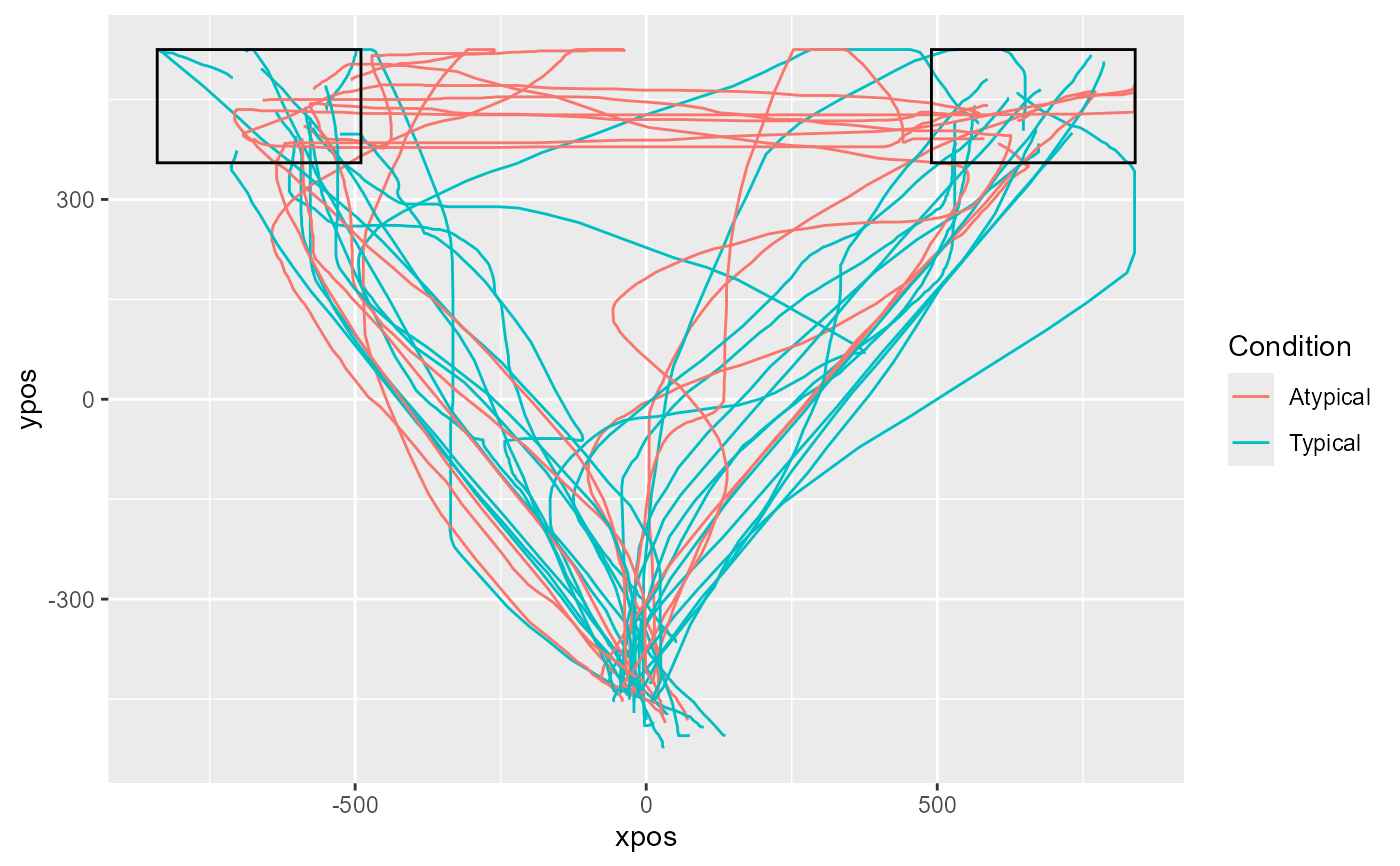mt_plot_add_rect adds one or several rectangles to a mousetrap plot. These buttons usually correspond to the borders of the buttons in the mouse-tracking experiment. It is specifically designed so that the arguments from the mousetrap_response plugin in OpenSesame can be used.

mt_plot_add_rect(rect, color = "black", fill = NA, ...)

## Arguments

rect

a data.frame or matrix with one row per box. For each rectangle, the x-position (x), y-position (y), width (w), and height (h) needs to be provided. If columns are not labeled, the order x, y, w, h is assumed.

color

argument passed on to geom_rect. Specifies the color of the border of the rectangles.

fill

argument passed on to geom_rect. Specifies the color of the interior of the rectangles. If NA (the default), rectangles are unfilled.

...

additional arguments passed on to geom_rect.

## Details

mt_plot_add_rect internally uses geom_rect of the ggplot2 package for plotting.

mt_plot for plotting trajectory data.

## Author

Pascal J. Kieslich

Felix Henninger

## Examples

# Load ggplot2
library(ggplot2)

# Import, flip, and time-normalize raw trajectories
mt_example <- mt_import_mousetrap(mt_example_raw)
mt_example <- mt_remap_symmetric(mt_example,remap_xpos="no")
mt_example <- mt_time_normalize(mt_example)

# Create rectangles matrix
rectangles <- matrix(
# (The matrix is n x 4, and contains
# all relevant data for every button,
# (i.e. x, y, width and height values)
# in separate rows)
c(
-840, 525,  350, -170,
840, 525, -350, -170
),
ncol=4, byrow=TRUE)

# Plot all time-normalized trajectories
# varying the color depending on the condition